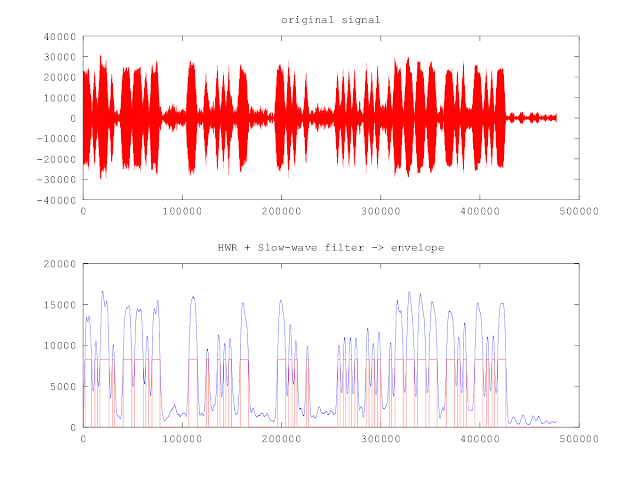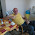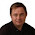Sunday, April 15, 2012

Experiment: Decoding multiple Morse code signals automatically on a noisy RF bandLibrary of Congress, Prints & Photographs Division, FSA-OWI Collection
While experienced CW operators can easily copy morse code from multiple stations in a pile-up even on a noisy RF band,  writing software to enable computers to accomplish similar task is quite difficult. CW Skimmer is an example of such software and it has gained popularity and some good reviews. However, CW Skimmer is not open source software and it has some limitations.

Rob Frohne, KL7NA has provided both excellent papers on the topic (1,2) as well as  working code examples to create, detect and decode morse code from noisy audio signals. I wanted to experiment and expand Rob's software written for Octave, open source software similar to Matlab.  I have been using Octave v3.4.2 on Ubuntu V11.1 running on my Thinkpad T43 laptop.

In order to do some experiments  and learn more how this problem could be solved I decided to break the problem into 4 different tasks, namely the following:

1)  Create a test case with 9 simultaneous morse code signals in different frequencies with noise.
2)  Create an algorithm to find all morse signals by frequency.
3)  Utilize  Rob's  matched filter code to improve signal to noise on selected frequency.
4)  Apply morse code decoding algorithm to filtered signals.

1)  CREATING A TEST CASE

I used Rob's morse.m  version to create a test audio file simulating a snapshot from a contest.  The code below simulates 9 stations with different call signs in various phases sending "CQ TEST DE  XXXXX"  with different speeds on different audio frequencies. These signals are then scaled and added up to create roughly S1...S9 signals between 400Hz and 1200Hz. Resulting 10 second long audio signal is then saved on 'cwcombo.wav' file.

function morse_file()
% create a test audio file with multiple morse stations in a pile-up

Fs = 48000; % Fs is sampling frequency - 48 Khz
Ts = 10*Fs; % Total sample time is 10 seconds

% create 9 different parallel morse sessions - 10 seconds each at 20-35 WPM speed
%         TEXT         audio file  noiselevel Hz    speed WPM
x1=morse('CQ TEST DE AG1LE','cw1.wav', 0.03125,1200,Fs,20, Ts);
x2=morse('TEST DE SP3RQ CQ','cw2.wav', 0.0625, 1100,Fs,35, Ts);
x3=morse('DE W3RQS CQ TEST','cw3.wav', 0.125, 1000,Fs,30, Ts);
x4=morse('SM0LXW CQ TEST DE','cw4.wav',0.25, 900,Fs, 25, Ts);
x5=morse('CQ TEST DE HS1DX','cw5.wav', 0.5, 800,Fs, 20, Ts);
x6=morse('TEST DE JA1DX CQ','cw6.wav', 1, 700,Fs, 20, Ts);
x7=morse('DE JA2ATA CQ TEST','cw7.wav',2, 600,Fs, 20, Ts);
x8=morse('UA2HH CQ TEST DE','cw8.wav', 4, 500,Fs, 20, Ts);
x9=morse('CQ TEST DE CT1CX','cw9.wav', 8, 400,Fs, 20, Ts);

% weighted sum - merge all the audio streams together
% 2x signal strength corresponds to 6 dB (one S-unit)
% 9 signals arranged S9 to S1 in frequency order 1200Hz ... 400Hz
y = 256*x1 + 128*x2 + 64*x3 + 32*x4 + 16*x5 + 8*x6 + 4*x7 + 2*x8 + x9;

% write to cwcombo.wav file
wavwrite(y,Fs,'cwcombo.wav');

% look at the spectrum of combined signals - display 100Hz...2500Hz
spektri(y,Fs,100,2500);

endfunction;

2) ALGORITHM TO FIND MORSE SIGNALS BY FREQUENCY

I created a small function "spektri"  to calculate FFT (fast fourier transform) and plot the audio spectrum in a given frequency range. The input is the above 10 second combined audio clip. The resulting spectrum is shown in Figure 1. below. The 9 simulated "stations" are 100 Hz apart from each other. Noise level is at around 90 dB and the  1200Hz  peak signal is at about 144 dB.  The range is about 54 dB corresponding to scale of roughly 9  S units if this signal would be coming from a real ham radio.  Signal x1 would peak at S9 and signal x9 would be S1 at almost noise level.Figure 1.  Morse code  test case - spectrum 100 - 2500 Hz.

Visually it is relatively easy to find the 7 to 8 strongest peaks from the picture above. However, finding those frequency peaks automatically is more challenging due to noise and some splatter components.

After testing various peak detection algorithms I found the following that seems to work quite well:

function [maxtab, mintab]=peakdet(v, delta, x)
%PEAKDET Detect peaks in a vector
%        [MAXTAB, MINTAB] = PEAKDET(V, DELTA) finds the local
%        maxima and minima ("peaks") in the vector V.
%        MAXTAB and MINTAB consists of two columns. Column 1
%        contains indices in V, and column 2 the found values.
%
%        With [MAXTAB, MINTAB] = PEAKDET(V, DELTA, X) the indices
%        in MAXTAB and MINTAB are replaced with the corresponding
%        X-values.
%
%        A point is considered a maximum peak if it has the maximal
%        value, and was preceded (to the left) by a value lower by
%        DELTA.

% Eli Billauer, 3.4.05 (Explicitly not copyrighted).
% This function is released to the public domain; Any use is allowed.

maxtab = [];
mintab = [];

v = v(:); % Just in case this wasn't a proper vector

if nargin < 3
x = (1:length(v))';
else
x = x(:);
if length(v)~= length(x)
error('Input vectors v and x must have same length');
end
end

if (length(delta(:)))>1
error('Input argument DELTA must be a scalar');
end

if delta <= 0
error('Input argument DELTA must be positive');
end

mn = Inf; mx = -Inf;
mnpos = NaN; mxpos = NaN;

lookformax = 1;

for i=1:length(v)
this = v(i);
if this > mx, mx = this; mxpos = x(i); end
if this < mn, mn = this; mnpos = x(i); end

if lookformax
if this < mx-delta
maxtab = [maxtab ; mxpos mx];
mn = this; mnpos = x(i);
lookformax = 0;
end
else
if this > mn+delta
mintab = [mintab ; mnpos mn];
mx = this; mxpos = x(i);
lookformax = 1;
end
end
end

I created another function that plots the spectrum and detected peaks on the same graph using the peak detection function above.

function  fpeaks = spektri_p(data, Fs, str_f, stp_f, delta);
% plot spectrum of data
% Fs = sampling frequency (48000)
% str_f = start frequency range to plot
% stp_f = stop frequency range to plot
% delta  =  A point is considered a maximum peak if it has the maximal
%        value, and was preceded (to the left) by a value lower by delta

N = length(data);
spec = fft(data); % do fourier transform
df = Fs/N; % frequency bin size
minf = -Fs/2;
maxf = Fs/2 -df;
i = round(N/2+(str_f*N/2)/(Fs/2)); % start index of freq range
j = round(N/2+(stp_f*N/2)/(Fs/2)); % stop index of freq range

f = [minf:df:maxf]; % frequency axis i.e. [ -24kHz...+24kHz ]
y = 20*log10(abs(fftshift(spec))); % dB magnitude
[maxtab,mintab] = peakdet(y(i:j,1),delta); % detect max peaks in range w/ delta dB difference
nr = length(maxtab(:,1))   % nr of peaks found

figure(2);
plot(f(1,i:j),y(i:j,1)); % plot audio spectrum within range
hold on;
plot(f(1,maxtab(:,1)+i),maxtab(:,2),'r*');  % plot found peaks within range
hold off;
title('Spectrum');
xlabel('Frequency(Hz)');
ylabel('Volume dB');
fpeaks = f(1,maxtab(:,1)+i)'; % return found frequency peaks within range

Using above plotting function pks = spektri_p(y,Fs,300,1300,45);
creates the following figure 2. Adjusting the last parameter (delta) will increase/decrease the number of peaks detected. Peaks are marked with red stars in the graph.Figure 2.  Frequency peaks marked with red star

Looking at the result  with delta = 45  this algorithm detects all peaks correctly within few Hz accuracy.
The pks variable contains the detected values, see below:

pks =

308.30
400.00
500.00
600.00
700.00
799.90
895.70
999.80
1103.50
1199.90

If the program would be more interactive this parameter "delta" could be adjustable variable, similar to squelch function in some radios. Lowering this parameter value would work like squelch - more noise peaks would be detected. Increasing the value would  allow to detect only the strongest stations.

3) MATCHED FILTER TO IMPROVE SIGNAL-TO-NOISE RATIO

Now that we have automatically found the peak audio frequencies of the morse signals we can apply a matched filter to improve the signal-to-noise ratio.

Below is the matched filter algorithm. The parameters include the audio signal, estimated morse speed in WPM,  sampling frequency Fs, and audio frequency of the morse signal.

% This script shows how a matched filter (dot product) works.
% See http://en.wikipedia.org/wiki/Matched_filter for theory

function x_f = mfilter(x,speed,Fs,codef);
%x  is audio signal (read from wavfile)
%Fs=48000; sampling frequency
%speed = 20; morse code speed in WPM
%code_f = 440; morse code audio frequency

dit_time = 1.2/speed;
x_length = length(x);

t=0:1/Fs:dit_time;

burst = sin(2*pi*codef*t);   % template of a "dit"
N = length(burst);

for k=1:x_length-N
xk = x(k:1:(k+N-1)); % time reversed signal
x_f1(k) = burst*xk;  % dot (inner) product with template
end
x_f = x_f1; %return filtered signal

Matched filter produces pretty dramatic effect - you can see it from the "before and after"  figure 3  below.
All the graphs show the actual audio signals - 480,000 samples over 10 second period.

Red graph shows the original 10 seconds audio clip produced by this line of code:
x6=morse('TEST DE JA1DX CQ','cw6.wav', 1, 700,Fs, 20, Ts);
Morse signal is barely visible from the noise.

Blue  graph shows the combined 10 second audio clip produced by this line of code:
y=256*x1+128*x2+64*x3+32*x4+16*x5+8*x6+4*x7+2*x8+x9;
The  strong S9 signal x1 dominates and the morse signal is clearly visible. Can you read the morse code produced by this line of code below by looking at the blue signal alone? x1=morse('CQ TEST DE AG1LE','cw1.wav', 0.03125,1200,Fs,20, Ts);
As there are 8 other signals embedded in the blue graph it is not as clean as in figure 4 where only x1 signal is visible.

Green  graph shows the output of  the matched filter:  y6 = mfilter(y,20,Fs,700).
The matched filter extracts from the combined audio the original noisy S4 level x6 signal  at 700Hz and using the morse code template "dit"  makes the original morse code visible.   Can you read 'TEST DE JA1DX CQ' by looking at green graph alone? There are some extra noise peaks making it more difficult.Figure 3. Matched Filter  demonstration

By using the results from section 2 above we can now run the matched filter on all peak frequencies where we found morse signals. This way we can extract automatically each "station" for the final morse code decoding step.

One area that is still open here is the morse speed. The speed is an essential parameter for the matched filter to create a "dit" template with proper duration  for the dot product.  In this example above we used a known speed. In real life morse speed has to be estimated. Once a few proper  "dits" and "dahs"  are received the speed estimation is pretty simple. Speed is  1.2 / dit_time. I have not implemented automatic speed tracking yet.

Matched filter has also some other interesting properties - more details in here.

4) MORSE CODE DECODING

Decoding morse code seems intuitively a pretty simple task, but when the signal has significant amount of noise present it becomes more difficult to produce reliable results.

Rob Frohne, KL7NA provides morse decoding software written for Octave in here.
The software reads the audio wavfile,  creates rectified and filtered morse signal and then detects short / long pulses, uses tokenized table lookup to decode morse code to text.

However,  Rob's version has some built-in assumptions about the audio signals.  He has a fixed threshold (0.05) and in the presence of noise this assumption can produce a lot of errors. I experimented with the threshold with many different kind of signals  and the following works a little bit better.

agc = max(y);
threshold = agc/2;

Proper AGC  (automatic gain control) would be needed if the signal strength varies a lot over time. Otherwise decoder will use incorrect threshold to determine when the signal starts and ends. This leads to incorrect timing of "dits" and "dahs".

Rob's  slow wave filter  (y = filter(ones(1,20)/20,1, x2);)  has also a fixed assumption of  the window size.   If the morse speed is very high the slow wave filter can smoothen fast "dits" too much.  On the other hand noise spikes need to be filtered, otherwise decoder misinterprets those as "dits".  There should be some mechanism to adjust filter window size based on morse speed.  I tried the following code that made some improvement. It requires  "speed" parameter though. This is a "chicken and egg" problem of the morse code  - you need to receive at least one "dit" and one "dah" to determine the actual speed. Starting from some reasonable default value like 20 WPM is also possible.

Fs =48000;
dit = 1.2 / speed;
dit_samples = Fs*dit;

flt = dit_samples;
y = filter(ones(1,flt)/flt,1, x2);

With relatively noise free signals this algorithm seems to work OK.
See figure 4. below for  S9  signal - original x1 version, produced by this line of code

x1=morse('CQ TEST DE AG1LE','cw1.wav', 0.03125,1200,Fs,20, Ts);
The upper red graph is the original signal - 480,000 samples over 10 seconds.  The bottom blue graph shows rectified & filtered envelope of the signal, and red overlapping line with threshold at 0.32 level determining "dits" and "dahs".Figure 4. Morse decoding process - original S9 signal

With somewhat noisy signals the matched filter helps - this S7 signal below in figure 5 is extracted using
y3 = mfilter(y,30,Fs,1000)  - text "DE W3RQS CQ TEST" is still readable from the bottom red graph below.Figure 5.  Morse decoding process - S7 signal y3 extracted with matched filter.

With  noisy signals the matched filter helps a lot - this S5 signal in figure 6 below is extracted using
y5 = mfilter(y,20,Fs,800)  - text "CQ TEST DE HS1DX" is still feasible to decode despite noise spikes.Figure 6. Morse decoding process - S5 signal y5 extracted with matched filter

With  noisy signals the matched filter helps but is not enough  - this S3 signal below is extracted using y7 = mfilter(y,20,Fs,600)  - text "DE JA2ATA CQ TEST" is missing some "dits". If threshold would be set lower then extra "dits" would be created from noise spikes. This will show up as errors in the decoding process.Figure 7. Morse decoding process - S3 signal y7 extracted with matched filter

Finally with signals buried under noise the matched filter helps but is not enough especially in pile-up or contest situation.  This S1 signal below is extracted using y9 = mfilter(y,20,Fs,400)  - text "CQ TEST DE CT1CX" is no longer decodeable. Human eye is good for pattern matching but noise distorts the signal below to almost unreadable.Figure 8. Morse decoding process - S1 signal  y9 extracted with matched filter

CONCLUSIONS

Detecting and decoding multiple morse signals simultaneously in a ham radio contest or pile-up situation on a noisy RF band  is not an easy task.  Even for experienced human operators it is hard to copy weak S1..S3 signals in the presence of strong S7...S9 signals.

With some advanced digital signal processing algorithms  it is possible to automatically detect multiple stations with S1...S9 signal strengths close to each others on a noisy RF band.  The algorithm presented in section 2 above will correctly determine peak signal frequencies for further processing.

Matched Filter has some unique properties making it suitable for extracting maximum signal-to-noise ratio for the signal of interest.  Using the matched filter for peak frequencies found with the algorithm above enables  automatic decoding of S5...S9 level signals virtually error free.  However, for the weaker S1...S3 level signals the presented method will produce errors.

On the decoding software some minor improvements were implemented, such as threshold determination based on overall signal level, as well as  slow wave filtering using morse code speed dependent filter windows. These minor improvements made the decoding software a bit more robust.

Experimenting with Gnu Octave software is an excellent way to gain insight on signal processing concepts and also to understand better the limitations of morse code in the presence of noise.

73 de AG1LE

PS. See also the latest experiment where above functionality was added on FLDIGI

1.Nice work, Mauri! Reminds me of my initial 1990's PhD research renewed recently with YT7PWR help. I work with Morse Runner simulator of HF contests. Life is easy until QRM is enabled. You might find more at Facebook or Googlecode.

73 de Mario, S56A, N1YU

2.Hi Mauri -- very interesting directions you are going. On your matched filter, you are assuming a sinewave burst, but depending on starting phase of received audio, it might be an arbitrary shift of the sine wave. So a better possible approach is the RSS of cross correlation against both a sinewave and a cosine wave (same as DFT). That keeps it detecting well, regardless of relative phase shift between signal and template.

- 73 de Dave, N7AIG

3.Hi David
Thanks for the tip. I did this experiment on very simple matched filter code using Octave (Matlab compatible open source language). Since I have well defined test audio files this approach works well. In real life the phase, strength and even frequency of morse code signals vary, not to mention speed variances. I have not tried DFT in Octave simulation but in the FLDIGI implementation there are two filtering options - one using frequency domain matched filter and another one using FFT filter (see http://www.w1hkj.com/FldigiHelp-3.21/configCW.html). In lower signal to noise situations matched filter seems to work a bit better.

- 73 de Mauri, AG1LE